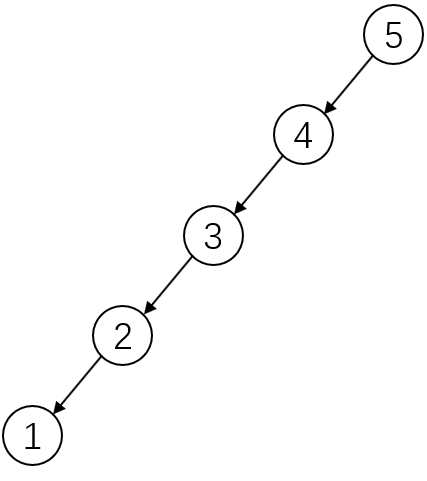# 二叉树的遍历算法

Posted by 江湖迈杰的博客 on December 25, 2017

# 二叉树的遍历算法

``````class TreeNode{
int val;
TreeNode left,right;
}
``````

# 树的递归遍历算法

``````void preOrder(TreeNode root){
if(root==null) return ;
System.out.println(root.val);
preOrder(root.left);
preOrder(root.right);
}
``````

``````void inOrder(TreeNode root){
if(root==null) return ;
inOrder(root.left);
System.out.println(root.val);
inOrder(root.right);
}
``````

``````void postOrder(TreeNode root){
if(root==null) return;
postOrder(root.left);
postOrder(root.right);
System.out.println(root.val);
}
``````

# 基于Stack 的实现方法

## 前序遍历

``````void preOrderWithStack(TreeNode root){
if(root==null) return ;
Stack<TreeNode> stack =new Stack();
stack.add(root);
while(!stack.isEmpty()){
TreeNode node = stack.pop();
System.out.print(node.val);
if(node.right!=null){
stack.push(node.right);
}
if(node.left!=null){
stack.push(node.left);
}
}
}
``````

## 中序遍历

### 一种简单的情况``````void inOrderWithStack(TreeNode root){
if(root==null) return;
Stack<TreeNode> stack = new Stack();
TreeNode node = root;
while(node!=null){ // ①查找root树的最小元素
stack.push(node);
node = node.left;
}
while(!stack.isEmpty()){ // ②依次弹出栈中的元素
node = stack.pop();
System.out.print(node.val);
}
}
``````

### 最终的代码实现

``````void inOrderWithStack(TreeNode root){
if(root==null) return ;
Stack<TreeNode> stack = new Stack();
TreeNode node = root;
while(!stack.isEmpty()||node!=null){
while(node!=null){ //①查找root树的最小元素
stack.push(node);
node = node.left;
}
node = stack.pop(); // ②弹出栈顶元素，并输出
System.out.print(node.val);
node =node.right; // ③node指向右子树
}
}
``````

## 后序遍历

### 比较简单的方法

``````void postOrderWithStack(TreeNode root){
if(root==null) return ;
Stack<Integer> result = new Stack();
Stack<TreeNode> stack = new Stack();
stack.add(root);
while(!stack.isEmpty()){
TreeNode node = stack.pop();
result.add(node.val);
if(node.left!=null){
stack.push(node.left);
}
if(node.right!=null){
stack.push(node.right);
}
}
while(!result.isEmpty()){
int val = result.pop();
System.out.print(val);
}
}
``````

### 另一种实现方式

``````void postOrderWithStack(TreeNode root){
if(root==null) return;
Stack<TreeNode>  stack = new Stack();
TreeNode = node ;
while(node!=null){
stack.push(node);
node=node.left;
}
while(!stack.isEmpty()){
node = stack.pop();
System.out.print(node.val);
}
}
``````

``````void postOrder(TreeNode root){
if(root==null) return;
TreeNode node = root;
TreeNode pre = null;
Stack<TreeNode> stack = new Stack<>();
while(!stack.isEmpty()||node!=null){
while(node!=null){
stack.push(node);
node = node.left;
}
node = stack.peek();
if(node.right ==null ||node.right==pre){ // 右子树为空或者右子树已经输出
System.out.println(node.val);
node = stack.pop();
pre = node;
node =null; // 防止重新进入循环
} else {
node = node.right;
}
}
}
``````